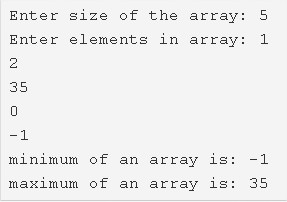# C Program To Find Maximum & Minimum Element In Array | C Prorams

C program to find the maximum and minimum element in an array – In this article, we will brief in on the many ways to find the maximum and minimum element in an array in C programming.

Suitable examples and sample programs have also been added so that you can understand the whole thing very clearly. The compiler has also been added with which you can execute it yourself.

The ways used in this piece are as follows:

• Using Standard Method
• Using Function
• Using Recursion

As we all know, arrays are a collection of a bunch of elements in a sequential pattern in a horizontal direction. Arrays form a very important of C programming.As given in the example above, firstly, enter the size of the array that you want to define.

The size of the array in the example mentioned is 5.

After that, you need to enter the elements of the array.

The elements entered in the array are as follows:

1 2 35 0 -1

So, the minimum of the array is -1 and the maximum of the array is 35.

Thus, doing the same using multiple methods in C programming is as follows:

## Using Standard Method

1. Read the entered array size and store that value into the variable n.

2) Read the entered elements using scanf and store the entered array elements into the array using for loop for(i=0;i<n;i++).

3) Initialise min, max values with the 1st element of the array.

4) Compare min, max values with a[i],

If min value is greater than a[i] then initialise min=a[i] and if max value is less than a[i] then initialise max=a[i]. Repeat this step for each element of the string using for loop which is having the structure for(i=1;i<n;i++).

Print the minimum of the array and maximum of the array values.

Output:

### Using Function

1. A function is a group of statements which perform a particular task. In this program sumofarray() is a function which finds the minimum and maximum of an array.

2) The main() function calls the sumofarray() function by passing an array, size of the array value as arguments.

3) The function sumofarray() compares the min, max values with array elements and prints the minimum of array element and maximum of array element values.

Output:

#### Using Recursion

1. A function which calls itself until some condition is called recursive function.

2) In this program, we have two recursive functions available.one is minimum() and another one is maximum(). Both these functions call by itself.

3) The main() function calls the minimum() by passing array,array size,1 as arguments.

Then the function minimum()

a) Checks the condition i<n, If it is true

b) Then it compares a[min]>a[i] if it is also true

c) Then min initialised to i and calls the function by itself by increasing i value until the condition a[min]>a[i] becomes false. This function returns the min to the main function.

main() function prints the a[min] value of the array.

4) The main() function calls the maximum() function by passing array,array size,1 as arguments.

Then the function maximum()

a) Checks the condition i<n, if it is true

b) Then it compares a[max]<a[i] if it is true

c) Then max initialise to i and calls the function itself by increasing i value until the condition a[max]<a[i] becomes false. This function returns the max to the main function.

main() function prints the a[max] value of the array.

Output:

x

## C Program To Print Number Of Days In A Month | Java Tutoring

C program to input the month number and print the number of days in that ...